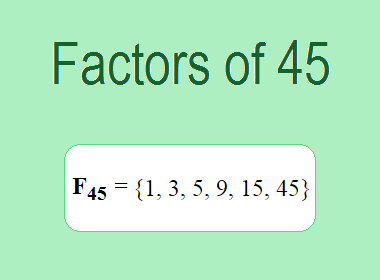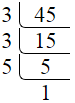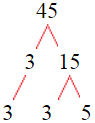# Factors of 45The factors of 45 are 1, 3, 5, 9, 15, and 45 i.e. F45 = {1, 3, 5, 9, 15, 45}. The factors of 45 are all the numbers that can divide 45 without leaving a remainder.

We can check if these numbers are factors of 45 by dividing 45 by each of them. If the result is a whole number, then the number is a factor of 45. Let's do this for each of the numbers listed above:

·        1 is a factor of 45 because 45 divided by 1 is 45.

·        3 is a factor of 45 because 45 divided by 3 is 15.

·        5 is a factor of 45 because 45 divided by 5 is 9.

·        9 is a factor of 45 because 45 divided by 9 is 5.

·        15 is a factor of 45 because 45 divided by 15 is 3.

·        45 is a factor of 45 because 45 divided by 45 is 1.

## How to Find Factors of 45?

1 and the number itself are the factors of every number. So, 1 and 45 are two factors of 45. To find the other factors of 45, we can start by dividing 45 by the numbers between 1 and 45. If we divide 45 by 2, we get a remainder of 1. Therefore, 2 is not a factor of 45. If we divide 45 by 3, we get a remainder of 0. Therefore, 3 is a factor of 45.

Next, we can check if 4 is a factor of 45. If we divide 45 by 4, we get a remainder of 1. Therefore, 4 is not a factor of 45. We can continue this process for all the possible factors of 45.

Through this process, we can find that the factors of 45 are 1, 3, 5, 9, 15, and 45. These are the only numbers that can divide 45 without leaving a remainder.

********************

********************

## Properties of the Factors of 45

The factors of 45 have some interesting properties. One of the properties is that the sum of the factors of 45 is equal to 78. We can see this by adding all the factors of 45 together:

1 + 3 + 5 + 9 + 15 + 45 = 78

Another property of the factors of 45 is that the prime factors of 45 are 3 and 5 only.

## Applications of the Factors of 45

The factors of 45 have several applications in mathematics. One of the applications is in finding the highest common factor (HCF) of two or more numbers. The HCF is the largest factor that two or more numbers have in common. For example, to find the HCF of 45 and 30, we need to find the factors of both numbers and identify the largest factor they have in common. The factors of 45 are 1, 3, 5, 9, 15, and 45. The factors of 30 are 1, 2, 3, 5, 6, 10, 15, and 30. The largest factor that they have in common is 15. Therefore, the HCF of 45 and 30 is 15.

Another application of the factors of 45 is in prime factorization. Prime factorization is the process of expressing a number as the product of its prime factors. The prime factors of 45 are 3 and 5 only. Therefore, we can express 45 as:

45 = 3 × 3 × 5

We can do prime factorization by division and factor tree method also. Here is the prime factorization of 45 by division method,45 = 3 × 3 × 5

Here is the prime factorization of 45 by the factor tree method,45 = 3 × 3 × 5

## Conclusion

The factors of 45 are the numbers that can divide 45 without leaving a remainder. The factors of 45 are 1, 3, 5, 9, 15, and 45. The factors of 45 have some interesting properties, such as having a sum of 78. The factors of 45 have several applications in mathematics, such as finding the highest common factor and prime factorization.